Home/Blog/Crack the top 40 machine learning interview questions

# Crack the top 40 machine learning interview questions

Oct 13, 2020 - 22 min read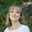Amanda Fawcett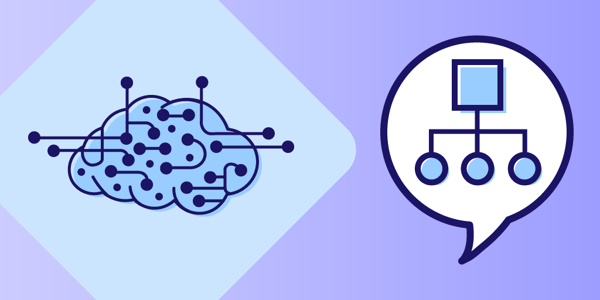Machine learning (ML) is the future of our world. In years to come, nearly every product will include ML components. ML is projected to grow from $7.3B in 2020 to$30.6B in 2024. This demand for ML skills is pervasive across the industry.

The machine learning interview is a rigorous process where candidates are assessed both for their knowledge of basic concepts and for understanding of ML systems, real-world applications, and product-specific demands.

If you are looking for a career in machine learning, it is crucial to understand what is expected in the interview. So, to help you prepare, I have collected the top 40 machine learning interview questions. We will begin with some of the basics and then move to advanced questions.

Today we will go over:

## Machine learning interview overview

Machine learning interview questions are an integral part of becoming a data scientist, machine learning engineer, or data engineer. Depending on the company, the job description title for a Machine Learning engineer may differ. You can expect to see titles like Machine Learning Engineer, Data Scientist, AI Engineer, and more.

Companies hiring for machine learning roles conduct interviews to assess individual abilities in various areas. ML interview questions tend to fall into one of these four categories.

• Algorithms and ML theory: How algorithms compare, how to measure them accurately
• Programming skills: Usually Python or domain-specific languages
• Interest in machine learning: Industry trends and your vision for ML components of the future
• Industry or product specific questions: How you take general ML knowledge and apply it to specific products

ML interview questions now focus heavily on system design. In the ML system design interview portion, candidates are given open-ended ML problems and are expected to build an end-to-end machine learning system. Common examples are recommendation systems, visual understanding systems, and search-ranking systems.## Company specific processes

Before we jump into the top 40 machine learning interview questions, let’s first take a look at how the top companies differ in their interview focuses.

### Google ML Interview

The Google ML interview, commonly called the Machine Learning Engineer interview, emphasizes skills in Algorithms, Machine Learning, and Python.

Some common questions include gradient descent, regularization/normalization methods, and embeddings.

The interview process will be generic rather than focused on one particular team or project. Once you pass the interview, they will assign you to a team that fits your skill set.

### Amazon ML Interview

The Amazon ML interview, called the Machine Learning Engineer Interview, focuses heavily on e-commerce ML tools, cloud computing, and AI recommendation systems.

Amazon ML engineers are expected to build ML systems and use Deep Learning models. Data scientists bridge data-driven gaps between the technical and business sides. Research scientists have higher levels of education and work to improve ASR, NLU, and TTS features.

The technical portion of the ML interview focuses on ML models, bias-variance tradeoff, and overfitting.

### Facebook ML Interview

The Facebook ML Interview consists of generic algorithm questions, ML design, and system design. You’ll be expected to work with newsfeed ranking algorithms and local search rankings. Facebook looks for engineers who understand components of an end-to-end ML system, including deployment.

Some common interview titles you may encounter are Research Scientist, Data Science Interview, or Machine Learning Engineer. Like Amazon, they differ slightly in their focus and demand for generalist knowledge.

### Twitter ML Interview

The data scientist roles at Twitter includes both data and research scientists roles that are each tailored to different teams.

The technical portion of interviews tests your application and intuition for ML theory (including SQL and Python). Twitter looks for knowledge of statistics, experimental models, product intuition, and system design.

## Beginner Questions (10)

Now let’s dive into the top 40 questions for an ML interview. These questions are broken into beginner, intermediate, advanced, and product specific questions.

### 1. What is the trade-off between bias and variance?

Bias (how well a model fits data) refers to errors due to inaccurate or simplistic assumptions in your ML algorithm, which leads to overfitting.

Variance (how much a model changes based on inputs) refers to errors due to complexity in your ML algorithm, which generates sensitivity to high levels of variation in training data and overfitting.

In other words, simple models are stable (low variance) but highly biased. Complex models are prone to overfitting but express the truth of the model (low bias). The optimal reduction of error requires a tradeoff of bias and variance to avoid both high variance and high bias.

### 2. Explain the difference between supervised and unsupervised machine learning.

Supervised learning requires training labeled data. In other words, supervised learning uses a ground truth, meaning we have existing knowledge of our outputs and samples. The goal here is to learn a function that approximates a relationship between inputs and outputs.

Unsupervised learning, on the other hand, does not use labeled outputs. The goal here is to infer the natural structure in a dataset.

### 3. What are the most common algorithms for supervised learning and unsupervised learning?

Supervised learning algorithms:

Examples of unsupervised algorithms:

• Clustering: k-Means
• Visualization and dimensionality reduction
• Principal component analysis (PCA), t-distributed
• Stochastic neighbor embedding (t-SNE)
• Association rule learning (Apriori)

### 4. Explain the difference between KNN and k-means clustering.

The main difference is that KNN requires labeled points (classification algorithm, supervised learning), but k-means does not (clustering algorithm, unsupervised learning).

To use K-Nearest Neighbors, you use labeled data that you want to classify into an unlabeled point. K-means clustering takes unlabeled points and learns how to group them using the mean of the distance between points.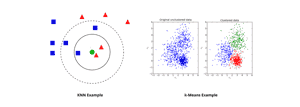### 5. What is the Bayes’ Theorem? Why do we use it?

Bayes’ Theorem is how we find a probability when we know other probabilities. In other words, it provides the posterior probability of a prior knowledge event. This theorem is a principled way of calculating conditional probabilities.

In ML, Bayes’ theorem is used in a probability framework that fits a model to a training dataset and for building classification predictive modeling problems (i.e. Naive Bayes, Bayes Optimal Classifier).

### 6. What are Naive Bayes classifiers? Why do we use them?

Naive Bayes classifiers are a collection of classification algorithms. These classifiers are a family of algorithms that share a common principle. Naive Bayes classifiers assume that the occurrence or absence of a feature does not influence the presence or absence of another feature.

In other words, we call this “naive”, as it assumes that all dataset features are equally important and independent.

Naive Bayes classifiers are used for classification. When the assumption of independence holds, they are easy to implement and yield better results than other sophisticated predictors. They are used in spam filtering, text analysis, and recommendation systems.

### 7. Explain difference between Type I and Type II error.

A Type I error is a false positive (claiming something has happened when it hasn’t), and a Type II error is a false negative (claiming nothing has happened when it actually has).

### 8. What is the difference between a discriminative and a generative model?

A discriminative model learns distinctions between different categories of data. A generative model learns categories of data. Discriminative models generally perform better on classification tasks.

### 9. What are parametric models? Provide an example.

Parametric models have a finite number of parameters. You only need to know the parameters of the model to make a data prediction. Common examples are as follows: linear SVMs, linear regression, and logistic regression.

Non-parametric models have an unbounded number of parameters to offer flexibility. For data predictions, you need the parameters of the model and the state of the observed data. Common examples are as follows: k-nearest neighbors, decision trees, and topic models.

### 10. Explain the difference between an array and a linked list.

An array is an ordered collection of objects. It assumes that every element has the same size, since the entire array is stored in a contiguous block of memory. The size of an array is specified at the time of declaration and cannot be changed afterward​.

Search options for an array are Linear search and Binary search (if it’s sorted).

A linked list is a series of objects with pointers. Different elements are stored at different memory locations, and data items can be added or removed when desired.

The only search option for a linked list is Linear.

Additional beginner questions may include:

• Which is more important: model performance or accuracy? Why?
• What’s the F1 score? How is it used?
• What is the Curse of Dimensionality?
• When should we use classification rather than regression?
• Explain Deep Learning. How does it differ from other techniques?
• Explain the difference between likelihood and probability.

## Intermediate Questions (15)

These intermediate questions take the basic theories of ML from above and apply them in a more rigorous way.

### 1. Which cross-validation technique would you choose for a time series dataset?

A time series is not randomly distributed but has a chronological ordering. You want to use something like forward chaining so you can model based on past data before looking at future data. For example:

• Fold 1 : training , test 
• Fold 2 : training [1 2], test 
• Fold 3 : training [1 2 3], test 
• Fold 4 : training [1 2 3 4], test 
• Fold 5 : training [1 2 3 4 5], test 

### 2. How do you choose a classifier based on a training set size?

For a small training set, a model with high bias and low variance models is better, as it is less likely overfit. An example is Naive Bayes.

For a large training set, a model with low bias and high variance models is better, as it expresses more complex relationships. An example is Logistic Regression.

### 3. Explain the ROC Curve and AUC.

The ROC curve is a graphical representation of the performance of a classification model at all thresholds. It has two thresholds: true positive rate and false positive rate.

AUC (Area Under the ROC Curve) is, simply, the area under the ROC curve. AUC measures the two-dimensional area underneath the ROC curve from (0,0) to (1,1). It used as a performance metric for evaluating binary classification models.### 4. Explain LDA for unsupervised learning.

Latent Dirichlet Allocation (LDA) is a common method for topic modeling. It is a generative model for representing documents as a combination of topics, each with their own probability distribution.

LDA aims to project the features of higher dimensional space onto a lower-dimensional space. This helps to avoid the curse of dimensionality.

### 5. How do you ensure you are not overfitting a model?

There are three methods we can use to prevent overfitting:

1. Use cross-validation techniques (like k-folds cross-validation)
2. Keep the model simple (i.e. take in fewer variables) to reduce variance
3. Use regularization techniques (like LASSO) that penalize model parameters likely to cause overfitting

### 6. In SQL, how are primary and foreign keys related?

SQL is one of the most popular data formats used in ML, so you need to demonstrate your ability to manipulate SQL databases.

Foreign keys allow you to match and join tables on the primary key of the corresponding table.

If you encounter this question, answer the basic concept, and the explain how you would set up SQL tables and query them.

### 7. What evaluation approaches would you use to gauge the effectiveness of an ML model?

First, you would split the dataset into training and test sets. You could also use a cross-validation technique to segment the dataset. Then, you would select and implement performance metrics. For example, you could use the confusion matrix, the F1 score and accuracy.

You’ll want to explain the nuances of how a model is measured based on different parameters. Interviewees that stand out take questions like these one step further.

### 8. Explain how to handle missing or corrupted data in a dataset.

You need to identify the find data and drop the rows/columns, or replace them with other values.

Pandas provides useful methods for doing this: isnull() and dropna(). These allow you to idenitfy and drop corrupted data. The fillna() method can be used to fill invalid values with placeholders.

### 9. Explain how you would develop a data pipeline.

Data pipelines enable us to take a data science model and automate or scale it. A common data pipeline tool is Apache Airflow, and Google Cloud, Azure, and AWS are used to host them.

For a question like this, you want to explain the required steps and discuss real experience you have building data pipelines.

The basic steps are as follows for a Google Cloud host:

2. Create a compute instance
3. Pull tutorial contents from GitHub
4. Use AirFlow for an overview of the pipeline
5. Use Docker to set up virtual hosts
6. Develop a Docker container
7. Open Airflow UI and run the ML pipeline
8. Run the deployed web app

### 10. How do you fix high variance in a model?

If the model has low variance and high bias, we use a bagging algorithm, which divides a data set into subsets using randomized sampling. We use those samples to generate a set of models with a single learning algorithm.

Additionally, we can use the regularization technique, in which higher model coefficients are penalized to lower the complexity overall.

### 11. What are hyperparameters? How do they differ from model parameters?

A model parameter is a variable that is internal to the model. The value of a parameter is estimated from training data.

A hyperparameter is a variable that is external to the model. The value cannot be estimated from data, and they are commonly used to estimate model parameters.

### 12.You are working on a dataset. How do you select important variables?

• Remove correlated variables before selecting important variables
• Use Random Forest and a plot variable importance chart
• Use Lasso Regression
• Use linear regression to select variables based on p values
• Use Forward Selection, Stepwise Selection, and Backward Selection

### 13. How do you choose which algorithm to use for a dataset?

Choosing an ML algorithm depends of the type of data in question. Business requirements are necessary for choosing an algorithm and building a is to build a model as well, so when answering this question, explain that you need more information.

For example, if you data organizes in a linear fashion, linear regression would be a good algorithm to use. Or, if the data is made up of non-linear interactions, a bagging or boosting algorithm is best. Or, if you’re working with images, a neural network would be best.

### 14. What are advantages and disadvantages of using neural networks?

• Store data on the entire network rather than a database
• Parallel processing
• Distributed memory
• Provides great accuracy even with limited information

• Requires complex processors
• Duration of a network is somewhat unknown
• We rely on error value too heavily
• Black-box nature

### 15. What is the default method for splitting in decision trees?

The default method is the Gini Index, which is the measure of impurity of a particular node. Essentially, it calculates the probability of a specific feature that is classified incorrectly. When the elements are linked by a single class, we call this “pure”.

You could also use Random Forest, but the Gini Index is preferred because it isn’t computationally intensive and doesn’t involve logarithm functions.

Additional intermediate questions may include:

• What is a Box-Cox transformation?
• Water Tapping problem
• Explain the advantages and disadvantages of decision trees.
• What is the exploding gradient problem when using back propagation technique?
• What is a confusion matrix? Why do you need it?

## Advanced Questions (10)

These advanced questions apply your knowledge to specific ML components and expand on the basic to think about real-world applications. These skills generally require coding rather than just theory.

### 1. You are given a data set with missing values that spread along 1 standard deviation from the median. What percentage of data would remain unaffected?

The data is spread across median, so we can assume we’re working with normal distribution. This means that approximately 68% of the data lies at 1 standard deviation from the mean. So, around 32% of the data unaffected.

### 2. You are told that your regression model is suffering from multicollinearity. How do verify this is true and build a better model?

You should create a correlation matrix to identify and remove variables with a correlation above 75%. Keep in mind that our threshold here is subjective.

You could also calculate VIF (variance inflation factor) to check for the presence of multicollinearity. A VIF value greater than or equal to 4 suggests that there is no multicollinearity. A value less than or equal to 10 tells us there are serious multicollinearity issues.

You can’t just remove variables, so you should use a penalized regression model or add random noise in the correlated variables, but this approach is less ideal.

### 3. Why does XGBoost perform better than SVM?

XGBoos is an ensemble method that uses many trees. This means it improves as it repeats itself.

SVM is a linear separator. So, if our data is not linearly separable, SVM requires a Kernel to get the data to a state where it can be separated. This can limit us, as there is not a perfect Kernel for every given dataset.

### 4. You build a random forest model with 10,000 trees. Training error as at 0.00, but validation error is 34.23. Explain what went wrong.

Your model is likely overfitted. A training error of 0.00 means that the classifier has mimicked training data patterns. This means that they aren’t available for our unseen data, returning a higher error.

When using random forest, this will occur if we use a large amount of trees.

### 5. Explain the stages for building an ML model.

This will largely depend on the model at hand, so you could ask clarifying questions. But generally, the process is as follows:

1. Understand the business model and end goal
2. Gather data acquisitions
3. Do data cleaning
4. Basic exploratory data analysis
5. Use machine learning algorithms to develop a model
6. Use an unknown dataset to check accuracy

### 6. What is the recall, specificity and precision of the confusion matrix below?

• TP / True Positive: the case was positive, and it was predicted as positive
• TN / True Negative: the case was negative, and it was predicted as negative
• FN / False Negative: the case was positive, but it was predicted as negative
• FP / False Positive: the case was negative, but it was predicted as positive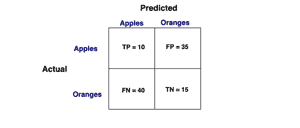• Recall = 20%
• Specificity = 30%
• Precision = 22%

Explanation:

Recall = TP / (TP+FN) = 10/50 = 0.2 = 20%

Specificity = TN / (TN+FP) = 15/50 = 0.3 = 30%

Precision = TP/ (TP + FP) = 10 / 45 = 0.2 = 22%

### 7. For NLP, what’s the main purpose of using an encoder-decoder model?

We use the encoder-decoder model to generate an output sequence based on an input sequence.

What makes an encoder-decoder model so powerful is that the decoder uses the final state of the encoder as its initial state. This gives the decoder access to the information that the encoder extracted from the input sequence.

### 8. For Deep Learning with TensorFlow, which value is required as an input to an evaluation EstimatorSpec?

The loss metric is required. In model execution with TensorFlow, we use the EstimatorSpec object to organize training, evaluation, and prediction.

The EstimatorSpec object is initialized with a single required argument, called mode. The mode can take one of three values:

• tf.estimator.ModeKeys.TRAIN
• tf.estimator.ModeKeys.EVAL
• tf.estimator.ModeKeys.PREDICT

The keyword arguments required to initialize the EstimatorSpec will differ depending on the mode.

### 9. When using scikit-learn, is it true that we need to scale our feature values when they vary greatly?

Yes. Most of the machine learning algorithms use Euclidean distance as the metrics to measure the distance between two data points. If the range of values is different greatly, the result of the same change in the different features will be very different.

### 10. Your dataset has 50 variables, but 8 variables have missing values higher than 30%. How do you address this?

There are three general approaches you could take:

1. Just remove them (not ideal)
2. Assign a unique category to the missing values to see if there is a trend generating this issue
3. Check distribution with the target variable. If a pattern is found, keep the missing values, assign them to a new category, and remove the others.

• You must evaluate a regression model based on R², adjusted R² and tolerance. What are your criteria?
• For k-means or kNN, why do we use Euclidean distance over Manhattan distance?
• Linear regression models are usually evaluated using Adjusted R² or an F value. How would you evaluate a logistic regression model?
• Explain the difference between the normal soft margin SVM and SVM with a linear kernel.

## Product-specific Questions (5)

Companies want to see that you can apply ML concepts to their real-world products and teams. You can expect questions about a company’s ML-based products and even be required to design them on your own.

### 1. How would you implement a recommendation system for our users?

Many ML interview questions like this involve implementing models to an organization’s specific problems. To answer this question well, you need to research the company in advance. Read about revenue drivers and user base.

Important: Use questions like these to demonstrate your system design skills! You need to sketch out a solution with requirements, metrics, training data generation, and ranking.

Grokking the Machine Learning Interview goes over this question in detail using Netflix’s recommendation system.

The general steps for setting up a recommendation system are as follows:

• Set up the problem by asking questions
• Understand scale and latency requirements
• Define the metrics for both online and offline testing
• Discuss the architecture of the system (how the data will flow)
• Discuss training data generation
• Outline feature engineering (what actors are involved)
• Discuss model training and algorithms
• Suggest how you’d scale and improve once it is deployed (i.e. issues you can predict)

### 2. What do you think is the most valuable data in our business?

This tests your knowledge of the business/industry. It also tests for how you correlated data to business outcomes and applies it to a particular company’s needs. You need to research an organization’s business model. Be sure to ask questions to clarify the question further before jumping in.

Some general answers could be:

• Quality data that is understood by ML teams is useful for scaling and making correct predictions
• Data that tells us what the customer wants is essential for all business decisions
• Better data management can increase their annual revenue
• The types of data most valuable to a company is customer data, IT data, and internal financial data

### 3. How would you structure the ad selection process for an ad prediction system?

The main goal of an ads selection component is to narrow down the set of ads that are relevant for a given query. In a search-based system, the ads selection component is responsible for retrieving the top relevant ads from the ads database according to the user and query context.

In a feed-based system, the ads selection component will select the top k relevant ads based more on user interests than search terms.

Here is a general solution to this question. Say we use a funnel-based approach for modeling. It would make sense to structure the ad selection process in these three phases:

• Phase 1: Quick selection of ads for the given query and user context according to selection criteria
• Phase 2: Rank these selected ads based on a simple and fast algorithm to trim ads.
• Phase 3: Apply the machine learning model on the trimmed ads to select the top ones.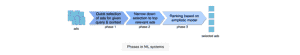### 4. What are the architectural components for a feed based system?

Again, this question largely depends on the organization in question. You’ll first want to ask clarifying questions about the system to make sure you meet all its needs. You can speak in hypotheticals to leave room for inaccuracy.

I will explain it using Twitter’s feed system to give you a sense of how to approach a problem like this. It will include:

• Tweet selection: a user’s pool of Tweets is forwarded to ranker components
• Training data generation: positive and negative training examples
• Ranker: For predicting probability of engagement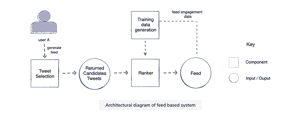### 5. What do you think about GPT-3? How do you think we can use it?

This question gauges your investment in the industry and you vision for how to apply new technologies. GPT-3 is a new language generation model that can generate human-like text.

There are many perspectives on GPT-3, so do some reading on how it’s being used to demonstrate next-generation critical thinking.

Some general answers could be:

• Improving chatbots and customer service automation
• Improving search engines with NLP
• Job training and presentations for ongoing learning
• Improving JSX code
• Simplifying UI/UX design

Additional questions could include:

• Design an ad prediction system for our company.
• What are the metrics for search ranking?
• What do you think of our current data process?
• Describe your research experience in machine learning.
• Write a query in SQL to measure the number of ads were viewed in moments versus news feed.
• How do you think quantum computing will affect ML at this organization?
• Which of our current products could benefit from ML components?

## What to learn next

Congrats! You’ve now learned the top 40 questions you will encounter in a machine learning interview. There is still a lot to learn to solidify your knowledge and get hands-on with system design, Python, and all the ML tools.

Be sure to review the additional questions I provided at the end of each section.

To move right into more practice, check out Educative’s course Grokking the Machine Learning Interview. You’ll learn how to design systems from scratch and develop a high-level ability to think about ML systems. This is the ideal place to take your ML skills to the next level and stand out from the competition.

Happy learning!